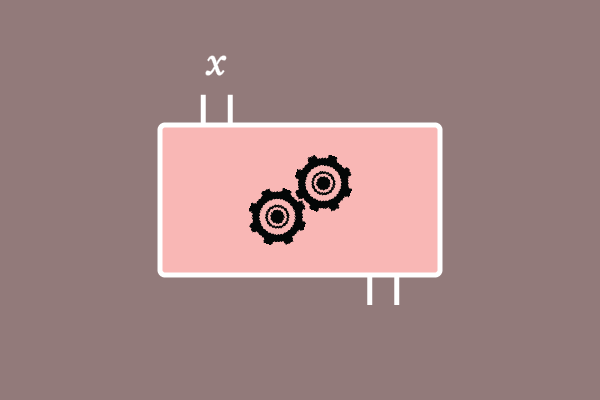# Function

Learn functions from basics to advanced level for all.

A mathematical relation of an element with another element and vice-versa is called a function.

### Introduction

Generally, any element can have a relation with another element. Similarly, a set of elements can also have a relation with another set of elements. A mathematical concept is introduced in mathematics to understand and represent the relation between them, and it is called a function.For example, $x$ is an element and it represents a quantity. Similarly, $y$ is an element and it also represents another quantity.

Assume, the element $x$ becomes $y$ by a relation between them. It happens in some cases. The mechanism of transformation of an element as another element is called a function.

The relation of two elements can be studied by the mathematics.

Latest Math Topics
May 11, 2020
May 02, 2020
Apr 30, 2020
Apr 29, 2020
Email subscription
Math Doubts is a best place to learn mathematics and from basics to advanced scientific level for students, teachers and researchers. Know more